Next: Takens-Theiler estimator Up: Dimensions and entropies Previous: Dimensions and entropies

## Correlation dimension

Roughly speaking, the idea behind certain quantifiers of dimensions is that the weight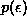of a typical-ball covering part of the invariant set scales with its diameter like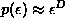, where the value for D depends also on the precise way one defines the weight. Using the square of the probability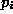to find a point of the set inside the ball, the dimension is called the correlation dimension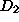, which is computed most efficiently by the correlation sum :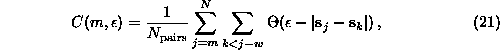where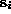are m-dimensional delay vectors,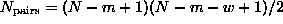the number of pairs of points covered by the sums,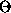is the Heaviside step function and w will be discussed below. On sufficiently small length scales and when the embedding dimension m exceeds the box-dimension of the attractor ,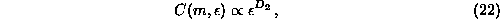Since one does not know the box-dimension a priori, one checks for convergence of the estimated values ofin m.

The literature on the correct and spurious estimation of the correlation dimension is huge and this is certainly not the place to repeat all the arguments. The relevant caveats and misconceptions are reviewed for example in Refs. [75, 11, 76, 2]. The most prominent precaution is to exclude temporally correlated points from the pair counting by the so called Theiler window w . In order to become a consistent estimator of the correlation integral (from which the dimension is derived) the correlation sum should cover a random sample of points drawn independently according to the invariant measure on the attractor. Successive elements of a time series are not usually independent. In particular for highly sampled flow data subsequent delay vectors are highly correlated. Theiler suggested to remove this spurious effect by simply ignoring all pairs of points in Eq.() whose time indices differ by less than w, where w should be chosen generously. With O(N²) pairs available, the loss of O(N) pairs is not dramatic as long as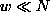. At the very least, pairs with j=k have to be excluded , since otherwise the strong bias to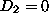, the mathematically correct value for a finite set of points, reduces the scaling range drastically. Choosing w, the first zero of the auto-correlation function, sometimes even the decay time of the autocorrelation function, are not large enough since they reflect only overall linear correlations [75, 76]. The space-time-separation plot (Sec.) provides a good means of determining a sufficient value for w, as discussed for example in [41, 2]. In some cases, notably processes with inverse power law spectra, inspection requires w to be of the order of the length of the time series. This indicates that the data does not sample an invariant attractor sufficiently and the estimation of invariants likeor Lyapunov exponents should be abandoned.

Parameters in the routines d2 and c2naive are as usual the embedding parameters m and, the time delay, and the embedding dimension, as well as the Theiler window.

Fast implementation of the correlation sum have been proposed by several authors. At small length scales, the computation of pairs can be done in O(NlogN) or even O(N) time rather than O(N²) without loosing any of the precious pairs, see Ref. . However, for intermediate size data sets we also need the correlation sum at intermediate length scales where neighbor searching becomes expensive. Many authors have tried to limit the use of computational resources by restricting one of the sums in Eq.() to a fraction of the available points. By this practice, however, one looses valuable statistics at the small length scales where points are so scarce anyway that all pairs are needed for stable results. In , buth approaches were combined for the first time by using fast neighbor search for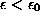and restricting the sum for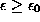. The TISEAN implementation d2 goes one step further and selects the range for the sums individually for each length scale to be processed. This turns out to give a major improvement in speed. The user can specify a desired number of pairs which seems large enough for a stable estimation of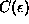, typically 1000 pairs will suffice. Then the sums are extended to a range which guarantees that number of pairs, or, if this cannot be achieved, to the whole time series. At the largest length scales, this range may be rather small and the user may choose to give a minimal number of reference points to ensure a representative average. In the program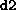, rather than restricting the range of the sums, only a randomly selected subset is used. The randomization however requires a sophisticated program structure in order to avoid an overhead in computation time.Next: Takens-Theiler estimator Up: Dimensions and entropies Previous: Dimensions and entropies

Thomas Schreiber
Wed Jan 6 15:38:27 CET 1999